# Zobel network

﻿
Zobel network
Linear analog electronic filters
edit
For the wave filter invented by Zobel and sometimes named after him see m-derived filters.

Zobel networks are a type of filter section based on the image impedance design principle. They are named after Otto Zobel of Bell Labs who published a much referenced paper on image filters in 1923. The distinguishing feature of Zobel networks is that the input impedance is fixed in the design independently of the transfer function. This characteristic is achieved at the expense of a much higher component count compared to other types of filter sections. The impedance would normally be specified to be constant and purely resistive. For this reason they are also known as constant resistance networks. However, any impedance achievable with discrete components is possible.

Zobel networks were formerly widely used in telecommunications to flatten and widen the frequency response of copper land lines, producing a higher quality line from one originally intended for ordinary telephone use. However, as analogue technology has given way to digital they are now little used.

When used to cancel out the reactive portion of loudspeaker impedance, the design is sometimes called a Boucherot cell. In this case, only half the network is implemented as fixed components, the other half being the real and imaginary components of the loudspeaker impedance. This network is more akin to the power factor correction circuits used in electrical power distribution, hence the association with Boucherot's name.

A common circuit form of Zobel networks is in the form of a bridged T. This term is often used to mean a Zobel network, sometimes incorrectly when the circuit implementation is, in fact, something other than a bridged T.BBC engineers equalising audio landlines circa 1959. The boxes with two large black dials towards the top of the equipment racks are adjustable Zobel equalisers. They are used both for temporary outside broadcast lines and for checking the engineer's calculations prior to building permanent units
Parts of this article or section rely on the reader's knowledge of the complex impedance representation of capacitors and inductors and on knowledge of the frequency domain representation of signals.

## Derivation

The basis of a Zobel network is a balanced bridge circuit as shown in the circuit to the right. The condition for balance is that;$\frac{Z}{Z_0}=\frac{Z_0}{Z'}.$

If this is expressed in terms of a normalised Z0 = 1 as is conventionally done in filter tables, then the balance condition is simply;$Z=\frac{1}{Z'}.$

In other words, Z' is simply the inverse, or dual impedance of Z.

The bridging impedance ZB is across the balance points and hence has no potential across it. Consequently, it will draw no current and its value makes no difference to the function of the circuit. However, its value is often chosen to be Z0 for reasons which will become clear in the discussion of bridged T circuits.

### Input impedance

The input impedance is given by$\frac{1}{Z_\text{in}}=\frac{1}{Z_0+Z'}+\frac{1}{Z+Z_0}.$

Substituting the balance condition,$Z'=\frac{Z_0^2}{Z},$

yields$Z_\text{in}=Z_0\,\!$

The input impedance can be designed to be purely resistive by setting$Z_0=R_0.\,$

The input impedance will then be real and independent of ω in band and out of band no matter what complexity of filter section is chosen.

### Transfer function

If the Z0 in the bottom right of the bridge is taken to be the output load then a transfer function of Vin/Vo can be calculated for the section. Only the rhs branch needs to be considered in this calculation. The reason for this can be seen by considering that there is no current flow through RB. None of the current flowing through the lhs branch is going to flow into the load. The lhs branch therefore, cannot possibly affect the output. It certainly affects the input impedance (and hence the input terminal voltage) but not the transfer function. The transfer function can now easily be seen to be;$A(\omega) = \frac{Z_0}{Z+Z_0}.$

### Bridged T implementation

The load impedance is actually the impedance of the following stage or of a transmission line and can sensibly be omitted from the circuit diagram. If we also set;$Z_B=Z_0\,\!$

then the circuit to the right results. This is referred to as a bridged T circuit because the impedance Z is seen to "bridge" across the T section. The purpose of setting ZB = Z0 is to make the filter section symmetrical. This has the advantage that it will then present the same impedance, Z0, at both the input and the output port.

## Types of section

A Zobel filter section can be implemented for low-pass, high-pass, band-pass or band-stop. It is also possible to implement a flat frequency response attenuator. This last is of some importance for the practical filter sections described later.

### Attenuator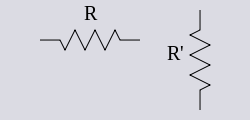Z and Z' for a Zobel attenuator

For an attenuator section, Z is simply$Z=R\,\!$

and,$Z'=R'=\frac{R_0^2}{R}$

The attenuation of the section is given by;$L=20\log\left(\frac{R}{R_0}+1\right) \, \text{dB}.$

### Low pass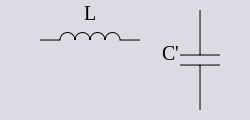Z and Z' for a Zobel low-pass filter section

For a low-pass filter section, Z is an inductor and Z' is a capacitor;$Z=i \omega L,\,$

and$Z'=\frac{1}{i \omega C'}$

where$C'=\frac{L}{R_0^2}.$

The transfer function of the section is given by$A(\omega)=\frac{R_0}{i \omega L+R_0}.$

The 3dB point occurs when ωL = R0 so the 3dB cut-off frequency is given by$\omega_c = \frac{R_0}{L},$

where ω is in the stop band well above ωc,$A(\omega) \approx \frac{R_0}{i \omega L},$

it can be seen from this that A(ω) is falling away in the stop band at the classic 6dB/8ve (or 20dB/decade).

### High pass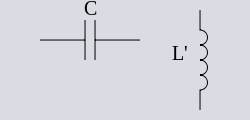Z and Z' for a Zobel high-pass filter section

For a high-pass filter section, Z is a capacitor and Z' is an inductor:$Z=\frac{1}{i \omega C},$

and$Z'=i \omega L'\,$

where$L'=CR_0^2.\,$

The transfer function of the section is given by$A(\omega)=\frac{i \omega C R_0}{1 + i \omega C R_0}.$

The 3dB point occurs when ωC = 1/R0 so the 3dB cut-off frequency is given by$\omega_c = \frac{1}{C R_0}.$

In the stop band,$A(\omega) \approx i \omega C R_0\,$

falling at 6dB/8ve with decreasing frequency.

### Band pass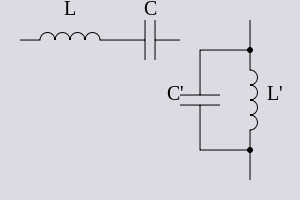Z and Z' for a Zobel band-pass filter section

For a band-pass filter section, Z is a series resonant circuit and Z' is a shunt resonant circuit;$Z=i \omega L + \frac{1}{i \omega C},$

and$Y'=\frac{1}{Z'}= i \omega C' + \frac{1}{i \omega L'}.$

The transfer function of the section is given by$A(\omega)=\frac{i \omega C R_0}{1 + i \omega C R_0 - \omega^2 L C}.$

The 3dB point occurs when |1 − ω2LC| = ωCR0 so the 3dB cut-off frequencies are given by$\omega_c = \frac{\pm R_0 C + \sqrt{R_0^2C^2+4 L C}}{2 L C},$

from which the centre frequency, ωm, and bandwidth, Δω, can be determined:$\Delta \omega = \frac{R_0}{L}$$\omega_m = \sqrt{\frac{R_0^2}{4 L^2}+\frac{1}{LC}}.$

Note that this is different from the resonant frequency$\omega_0 = \sqrt{\frac{1}{L C}};$

the relationship between them being given by$\omega_m^2 = \left(\frac{\Delta \omega}{2}\right)^2 +\omega_0^2.$

### Band stopZ and Z' for a Zobel band-stop filter section

For a band-stop filter section, Z is a shunt resonant circuit and Z' is a series resonant circuit:$Y=\frac{1}{Z}= i \omega C + \frac{1}{i \omega L},$

and$Z'=i \omega L' + \frac{1}{i \omega C'}.$

The transfer function and bandwidth can be found by analogy with the band-pass section.$\Delta\omega = \frac{1}{C R_0}.$

And,$\omega_m = \sqrt{\left(\frac{1}{2 R_0 C}\right)^2+\frac{1}{LC}}.$

## Practical sections

Zobel networks are rarely used for traditional frequency filtering. Other filter types are significantly more efficient for this purpose. Where Zobels come into their own is in frequency equalisation applications, particularly on transmission lines. The difficulty with transmission lines is that the impedance of the line varies in a complex way across the band and is tedious to measure. For most filter types, this variation in impedance will cause a significant difference in response to the theoretical, and is mathematically difficult to compensate for, even assuming that the impedance is known precisely. If Zobel networks are used however, it is only necessary to measure the line response into a fixed resistive load and then design an equaliser to compensate it. It is entirely unnecessary to know anything at all about the line impedance as the Zobel network will present exactly the same impedance to line as the measuring instruments. Its response will therefore be precisely as theoretically predicted. This is a tremendous advantage where high quality lines with flat frequency responses are desired.

### Basic loss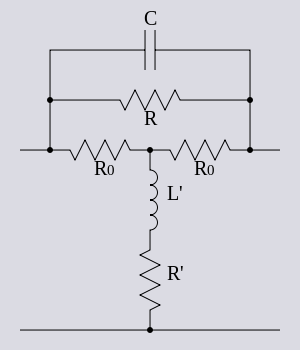A practical high pass section incorporating basic loss used to correct high end roll-off

For audio lines, it is invariably necessary to combine L/C filter components with resistive attenuator components in the same filter section. The reason for this is that the usual design strategy is to require the section to attenuate all frequencies down to the level of the frequency in the passband with the lowest level. Without the resistor components, the filter, at least in theory, would increase attenuation without limit. The attenuation in the stop band of the filter (that is, the limiting maximum attenuation) is referred to as the "basic loss" of the section. In other words, the flat part of the band is attenuated by the basic loss down to the level of the falling part of the band which it is desired to equalise. The following discussion of practical sections relates in particular to audio transmission lines.

### 6dB/octave roll-off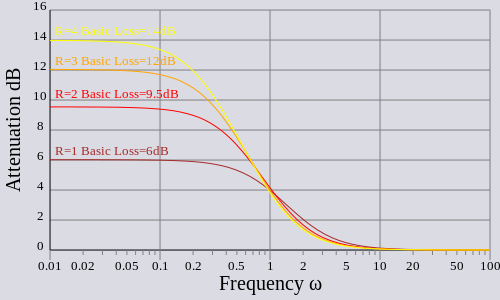High-pass Zobel network response for various basic losses. Normalised to R0 = 1 and ωc = 1

The most significant effect that needs to be compensated for is that at some cut-off frequency the line response starts to roll-off like a simple low-pass filter. The effective bandwidth of the line can be increased with a section that is a high-pass filter matching this roll-off, combined with an attenuator. In the flat part of the pass-band only the attenuator part of the filter section is significant. This is set at an attenuation equal to the level of the highest frequency of interest. All frequencies up to this point will then be equalised flat to an attenuated level. Above this point, the output of the filter will again start to roll-off.

### Mismatched lines

Quite commonly in telecomms networks, a circuit is made up of two sections of line which do not have the same characteristic impedance. For instance 150Ω and 300Ω. One effect of this is that the roll-off can start at 6dB/octave at an initial cut-off frequency fc1, but then at fc2 can become suddenly steeper. This situation then requires (at least) two high-pass sections to compensate each operating at a different fc.

### Bumps and dips

Bumps and dips in the passband can be compensated for with band-stop and band-pass sections respectively. Again, an attenuator element is also required, but usually rather smaller than that required for the roll-off. These anomalies in the pass-band can be caused by mismatched line segments as described above. Dips can also be caused by ground temperature variations.

### Transformer roll-offLow frequency equaliser section with compensation for inductor resistance. The resistance r represents the stray resistance of the non-ideal inductor. The resistance r' is a real resistor calculated to compensate for r.

Occasionally, a low-pass section is included to compensate for excessive line transformer roll-off at the low frequency end. However, this effect is usually very small compared to the other effects noted above.

Low frequency sections will usually have inductors of high values. Such inductors have many turns and consequently tend to have significant resistance. In order to keep the section constant resistance at the input, the dual branch of the bridge T must contain a dual of the stray resistance, that is, a resistor in parallel with the capacitor. Even with the compensation, the stray resistance still has the effect of inserting attenuation at low frequencies. This in turn has the effect of slightly reducing the amount of LF lift the section would otherwise have produced. The basic loss of the section can be increased by the same amount as the stray resistance is inserting and this will return the LF lift achieved to that designed for.

Compensation of inductor resistance is not such an issue at high frequencies were the inductors will tend to be smaller. In any case, for a high-pass section the inductor is in series with the basic loss resistor and the stray resistance can merely be subtracted from that resistor. On the other hand, the compensation technique may be required for resonant sections, especially a high Q resonator being used to lift a very narrow band. For these sections the value of inductors can also be large.

### Temperature compensation

An adjustable attenuation high-pass section can be used to compensate for changes in ground temperature. Ground temperature is very slow varying in comparison to surface temperature. Adjustments are usually only required 2-4 times per year for audio applications.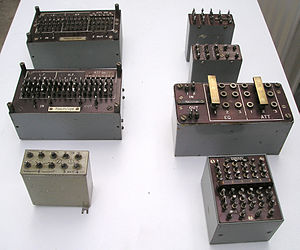A collection of various designs of temperature compensation equaliser. Some can be adjusted with plugable links, others require soldering. Adjustment is not very frequent.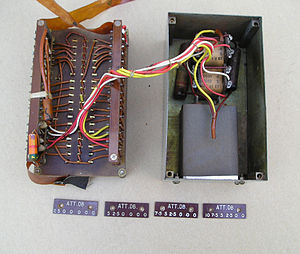The internal components of a temperature equaliser. The inductor and capacitor on the right set the frequency at which the equaliser starts to operate, the banks of selectable resistors on the left set the basic loss and hence amount of equalisation.

## Typical filter chain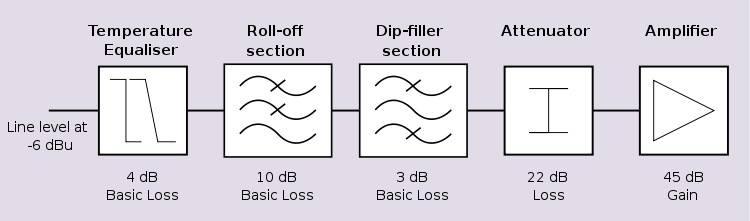An example of a typical chain of Zobel networks being used for line equalisation

A typical complete filter will consist of a number of Zobel sections for roll-off, frequency dips and temperature followed by a flat attenuator section to bring the level down to a standard attenuation. This is followed by a fixed gain amplifier to bring the signal back up to a usable level, typically 0dBu. The gain of the amplifier is usually no more than 45dB maximum. Any more and the amplification of line noise will tend to cancel out the quality benefits of improved bandwidth. This limit on amplification essentially limits how much the bandwidth can be increased by these techniques. It should also be noted that no one part of the incoming signal band will be amplified by the full 45dB. The 45dB is made up of the line loss in the flat part of its spectrum plus the basic loss of each section. In general, each section will be minimum loss at a different frequency band, hence the amplification in that band will be limited to the basic loss of just that one filter section, assuming insignificant overlap. A typical choice for R0 is 600 Ω. A good quality transformer (usually essential, but not shown on the diagram), known as a repeating coil, is at the beginning of the chain where the line terminates.

## Other section implementations

Besides the Bridged T, there are a number of other possible section forms that can be used.

### L-sections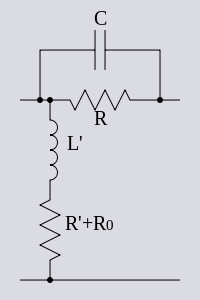Open circuit derived Zobel L-section for a high-pass section with basic loss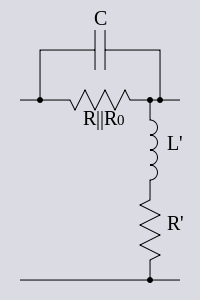Short circuit derived Zobel L-section for a high-pass section with basic loss

As mentioned above, ZB can be set to any desired impedance without affecting the input impedance. In particular, setting it as either an open circuit or a short circuit results in a simplified section circuit, called L–sections. These are shown above for the case of a high pass section with basic loss.

The input port still presents an impedance of R0 (provided that the output is terminated in R0) but the output port no longer presents a constant impedance. Both the open-circuit and the short-circuit L–sections are capable of being reversed so that R0 is then presented at the output and the variable impedance is presented at the input.

To retain the benefit of Zobel networks constant impedance, the variable impedance port must not face the line impedance. Nor should it face the variable impedance port of another half section. Facing the amplifier is acceptable since the input impedance of the amplifier is normally arranged to be R0 within acceptable tolerances. In other words, variable impedance must not face variable impedance.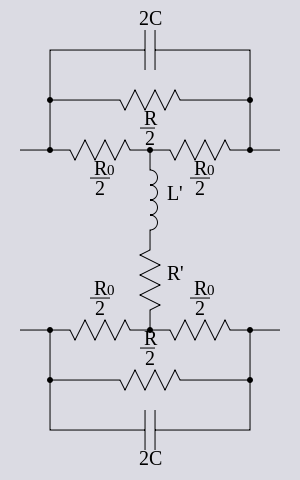A balanced bridged T high-pass full section with basic loss

### Balanced bridged T

The Zobel networks described here can be used to equalise land lines composed of twisted pair or star quad cables. The balanced circuit nature of these lines delivers a good common mode rejection ratio (CMRR). To maintain the CMRR, circuits connected to the line should maintain the balance. For this reason, balanced versions of Zobel networks are sometimes required. This is achieved by halving the impedance of the series components and then putting identical components in the return leg of the circuit.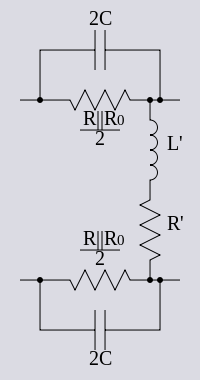A balanced Zobel high-pass short-circuit derived C-section with basic loss

### Balanced C-sections

A C–section is a balanced version of an L–section. The balance is achieved in the same way as a balanced full bridged T section by placing half of the series impedance in, what was, the common conductor. C–sections, like the L–section from which they are derived, can come in both open-circuit and short circuit varieties. The same restrictions apply to C–sections regarding impedance terminations as to L–sections.

### X-section

It is possible to transform a bridged–T section into a Lattice, or X–section. The X–section is a kind of bridge circuit, but usually drawn as a lattice, hence the name. Its topology makes it intrinsically balanced but it is never used to implement the constant resistance filters of the kind described here because of the increased component count. The component count increase arises out of the transformation process rather than the balance. There is however, one common application for this topology, the lattice phase equaliser, which is also constant resistance and also invented by Zobel. This circuit differs from those described here in that the bridge circuit is not generally in the balanced condition.

### Half sections

In respect of constant resistance filters, the term half section has a somewhat different meaning to other kinds of image filter. Generally, a half section is formed by cutting through the mid-point of the series impedance and shunt admittance of a full section of a ladder network. It is literally half a section. Here, however, there is a somewhat different definition. A half section is either the series impedance (series half-section) or shunt admittance (shunt half-section) that, when connected between source and load impedances of R0, will result in the same transfer function as some arbitrary constant resistance circuit. The purpose of using half sections is that the same functionality is achieved with a drastically reduced component count.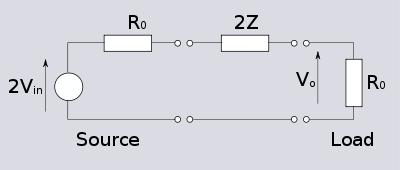A general Zobel series half section showing the equality of transfer function to an equivalent constant resistance section

If a constant resistance circuit has an input Vin, then a generator with an impedance R0 must have an open-circuit voltage of E=2Vin in order to produce Vin at the input of the constant resistance circuit. If now the constant resistance circuit is replaced by an impedance of 2Z, as in the diagram above, it can be seen by simple symmetry that the voltage Vin will appear half way along the impedance 2Z. The output of this circuit can now be calculated as,$\frac{V_O}{V_{in}}=\frac{R_0}{Z+R_0}$

which is precisely the same as a bridged T section with series element Z. The series half-section is thus a series impedance of 2Z. By corresponding reasoning, the shunt half-section is a shunt impedance of ½Z' (or twice the admittance).

It must be emphasised that these half sections are far from being constant resistance. They have the same transfer function as a constant resistance network, but only when correctly terminated. An equaliser will not give good results if a half-section is positioned facing the line since the line will have a variable (and probably unknown) impedance. Likewise, two half-sections cannot be connected directly to each other as these both will have variable impedances. However, if a sufficiently large attenuator is placed between the two variable impedances, this will have the effect of masking the effect. A high value attenuator will have an input impedance$\approx R_0$ no matter what the terminating impedance on the other side. In the example practical chain shown above there is a 22dB attenuator required in the chain. This does not need to be at the end of the chain, it can be placed anywhere desired and used to mask two mismatched impedances. It can also be split into two or more parts and used for masking more than one mismatch.

## Zobel networks and loudspeaker drivers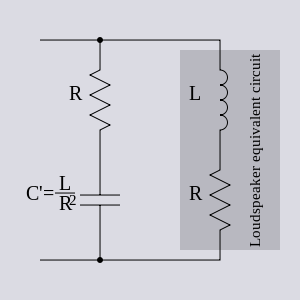Zobel network correcting loudspeaker impedance

Zobel networks can be used to make the impedance a loudspeaker presents to its amplifier output appear as a steady resistance. This is beneficial to the amplifier performance. The impedance of a loudspeaker is partly resistive. The resistance representing the energy transferred from the amplifier to the sound output plus some heating losses in the loudspeaker. However, the speaker also possesses inductance due to the windings of its coil. The impedance of the loudspeaker is thus typically modelled as a series resistor and inductor. A parallel circuit of a series resistor and capacitor of the correct values will form a Zobel bridge. It is obligatory to choose$R_B = \infin$ because the centre point between the inductor and resistor is inaccessible (and, in fact, fictitious - the resistor and inductor are distributed quantities as in a transmission line). The loudspeaker may be modelled more accurately by a more complex equivalent circuit. The compensating Zobel network will also become more complex to the same degree.

Note that the circuit will work just as well if the capacitor and resistor are interchanged. In this case the circuit is no longer a Zobel balanced bridge but clearly the impedance has not changed. The same circuit could have been arrived at by designing from Boucherot's minimising reactive power point of view. From this design approach there is no difference in the order of the capacitor and the resistor and Boucherot cell might be considered a more accurate description.

## Video equalisers

Zobel networks can be used for the equalisation of video lines as well as audio lines. There is, however, a noticeably different approach taken with the two types of signal. The difference in the cable characteristics can be summarised a s follows;

• Video commonly uses co-axial cable which requires an unbalanced topology for the filters whereas audio commonly uses twisted pair which requires a balanced topology.
• Video requires a wider bandwidth and tighter differential phase specification which in turn results in a tighter dimensional specification for the cable.
• The tighter specifications for video cable tends to produce a substantially constant characteristic impedance over a wide band (usually nominally 75 Ω). On the other hand, audio cable may be nominally 600 Ω (300 Ω and 150 Ω are also standard values), but it will only actually measure this value at 800 Hz. At a lower frequencies it will be much higher and at higher frequencies will be lower and more reactive.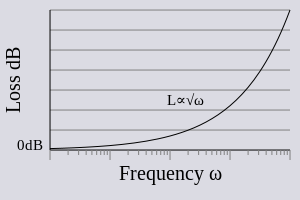Response plot of a video line showing the typical$\sqrt{f}$ response
• These characteristics result in a smoother, more well behaved response for video lines with none of the nasty discontinuities typically found with audio lines. These discontinuities in the frequency response are often caused by the habit of the telecom companies of forming a connection by joining two shorter lines of differing characteristic impedance. Video lines on the other hand tend to roll off smoothly with frequency in a predictable way.

This more predictable response of video allows a different design approach. The video equaliser is built as a single bridged T section but with a rather more complex network for Z. For short lines, or for a trimming equaliser, a Bode filter topology might be used. For longer lines a network with Cauer filter topology might be used. Another driver for this approach is the fact that a video signal occupies a large number of octaves, around 20 or so. If equalised with simple basic sections, a large number of filter sections would be required. Simple sections are designed, typically, to equalise a range of one or two octaves.

### Bode equaliser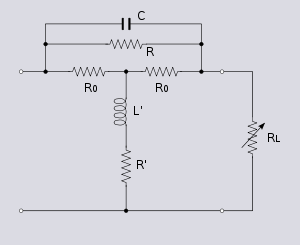Bode network for high frequency equalisation

A Bode network, as with a Zobel network, is a symmetrical bridge T network which meets the constant k condition. It does not however meet the constant resistance condition, that is, the bridge is not in balance. Any impedance network, Z, can be used in a Bode network, just as with a Zobel network, but the high pass section shown for correcting high-end frequencies is the most common. A Bode network terminated in a variable resistor can be used to produce a variable impedance at the input terminals of the network. A useful property of this network is that the input impedance can be made to vary from a capacitive impedance through a purely resistive impedance to an inductive impedance all by adjusting the single load potentiometer, RL. The bridging resistor, R0, is chosen to equal the nominal impedance so that in the special case when RL is set to R0 the network behaves as a Zobel network and Zin is also equal to R0.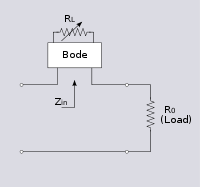Bode network used in an equaliser circuit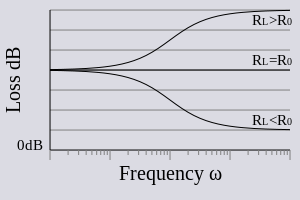Bode trimming equaliser response plot

The Bode network is used in an equaliser by connecting the whole network such that the input impedance of the Bode network, Zin, is in series with the load. Since the impedance of the Bode network can be either capacitive or inductive depending on the position of the adjustment potentiometer, the response may be a boost or a cut to the band of frequencies it is acting on. The transfer function of this arrangement is:$A(\omega)=\frac{R_0}{Z_{in}+R_0}$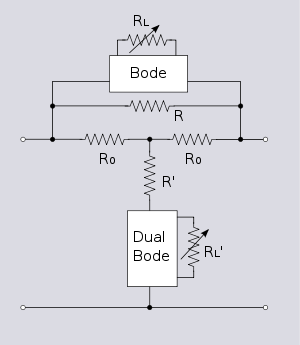Bode equaliser implemented as a Zobel constant resistance equaliser

The Bode equaliser can be converted into a constant resistance filter by using the entire Bode network as the Z branch of a Zobel network, resulting in a rather complex network of bridge T networks embedded in a larger bridge T. It can be seen that this results in the same transfer function by noting that the transfer function of the Bode equaliser is identical to the transfer function of the general form of Zobel equaliser. Note that the dual of a constant resistance bridge T network is the identical network. The dual of a Bode network is therefore the same network except for the load resistance RL, which must be the inverse, RL', in the dual circuit. To adjust the equaliser RL and RL' must be ganged, or otherwise kept in step such that as RL increases RL' will decrease and vice versa.

### Cauer equaliser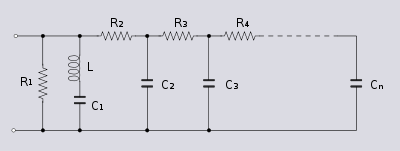Cauer topology network to be used as the Z impedance of a Zobel network equaliser

To equalise long video lines, a network with Cauer topology is used as the Z impedance of a Zobel constant resistance network. Just as the input impedance of a Bode network is used as the Z impedance of a Zobel network to form a Zobel Bode equaliser, so the input impedance of a Cauer network is used to make a Zobel Cauer equaliser. The equaliser is required to correct an attenuation increasing with frequency and for this a Cauer ladder network consisting of series resistors and shunt capacitors is required. Optionally, there may be an inductor included in series with the first capacitor which increases the equalisation at the high end due to the steeper slope produced as resonance is approached. This may be required on longer lines. The shunt resistor R1 provides the basic loss of the Zobel network in the usual way.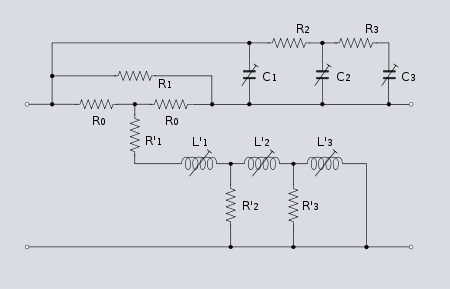Cauer Zobel bridge T implemented video equaliser. The impedance Z of this example consists of a three section ladder and is suitable for the equalisation of short lines (between nearby buildings for instance)

The dual of a RC Cauer network is a LR Cauer network which is required for the Z' impedance as shown in the example. Adjustment is a bit problematic with this equaliser. In order to maintain the constant resistance, the pairs of components C1/L1', C2/L2' etc., must remain dual impedances as the component is adjusted, so both parts of the pair must be adjusted together. With the Zobel Bode equaliser, this is a simple matter of ganging two pots together - a component configuration available off-the-shelf. Ganging together a variable capacitor and inductor is not, however, a very practical solution. These equalisers tend to be "hand built", one solution being to select the capacitors on test and fit fixed values according to the measurements and then adjust the inductors until the required match is achieved. The furthest element of the ladder from the driving point is equalising the lowest frequency of interest. This is adjusted first as it will also have an effect on higher frequencies and from there progressively higher frequencies are adjusted working along the ladder towards the driving point.

Wikimedia Foundation. 2010.

### Look at other dictionaries:

• Network synthesis filters — Network synthesis is a method of designing signal processing filters. It has produced several important classes of filter including the Butterworth filter, the Chebyshev filter and the Elliptic filter. It was originally intended to be applied to… …   Wikipedia

• Zobel — The original German term Zobel or its Spanish and Filipino renditions Zóbel is a surname, and may refer to:* Enrique Zobel * Fernando Zobel * Fernando Zobel de Ayala * Hiller B. Zobel Massachusetts judge and author * Jaime Augusto Zobel * Jaime… …   Wikipedia

• Otto Julius Zobel — Born October 20, 1887(1887 10 20) Ripon, Wisconsin Died January 1970 (age 82) Morristown, New Jersey …   Wikipedia

• Otto Julius Zobel — El estilo de esta traducción aún no ha sido revisado por terceros. Si eres hispanohablante nativo y no has participado en esta traducción puedes colaborar revisando y adaptando el estilo de ésta u otras traducciones ya acabadas. Otto Julius Zobel …   Wikipedia Español

• De La Salle-Santiago Zobel School — Infobox Secondary school name = native name = Paaralang De La Salle Santiago Zobel motto = Religio Mores Cultura Christian Achievers for God and Country established = 1978 city = Muntinlupa state = Metro Manila province = country = Philippines… …   Wikipedia

• De La Salle Santiago Zobel School — De La Salle–Santiago Zobel School Motto Religio Mores Cultura Christian Achievers for God and Country Established 1978 Type Coeducational, Lasallian, Catholic …   Wikipedia

• Joel Reyes Zobel — is a media personality of GMA Network s flagship AM station DZBB. He presently anchors Bangon na, Bayan! . He is also the co anchor of Mike Enriquez in the radio station s morning newscast Super Balita sa Umaga Nationwide and the voiceover of the …   Wikipedia

• Composite image filter — Linear analog electronic filters Network synthesis filters Butterworth filter Chebyshev filter Elliptic (Cauer) filter Bessel filter Gaussian filter Optimum L (Legendre) filter Linkwitz Riley filter …   Wikipedia

• Electronic filter topology — An elementary filter topology introduces a capacitor into the feedback path of an op amp to achieve an unbalanced active implementation of a low pass transfer function Electronic filter topology defines electronic filter circuits without taking… …   Wikipedia

• Constant k filter — Linear analog electronic filters Network synthesis filters Butterworth filter Chebyshev filter Elliptic (Cauer) filter Bessel filter Gaussian filter Optimum L (Legendre) filter Linkwitz Riley filter …   Wikipedia